# 基于立方体网格插值算法的气象雷达数据的三维重建3D Reconstruction of Meteorological Radar Data Based on Cubic Grid Interpolation Algorithm

• 全文下载: PDF(3340KB)    PP.1536-1545   DOI: 10.12677/CSA.2019.98172
• 下载量: 227  浏览量: 588   国家自然科学基金支持

In the meteorological field, meteorological objects have many targets, scattered regions, small areas, and irregular shapes in the whole detection range. Using conventional interpolation algorithms to reconstruct three-dimensional cloud image may result in low accuracy of image information and discrete and small object image information loss which makes the reconstructed three-dimensional cloud image data often not to meet the needs of real-time business. Based on the actual observation data of radar, this paper proposes and implements a cubic grid interpolation algorithm, which maps the irregular radar cone data into the regular 3D data field, and combines the Marching Cubes algorithm to perform 3D reconstruction experiments on meteorological radar data. The experimental results show that the 3D reconstruction cloud map obtained by the proposed algorithm can accurately reflect the basic characteristics and spatial distribution of meteorological targets, which meet the meteorological service requirements, and provide a reference for real-time tracking and accurate prediction of meteorological targets.

1. 引言

2. 方法描述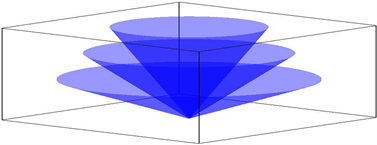Figure 1. Volume scanning diagram of meteorological radar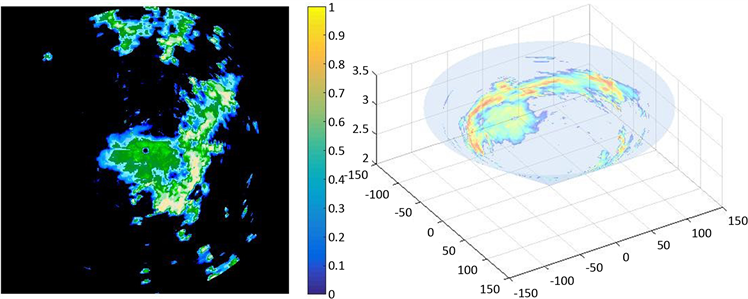(a) (b)

Figure 2. Radar echo intensity plane and the corresponding conical figure with a radar observation angle of 0.5 degree

2.1. 构建雷达锥体模型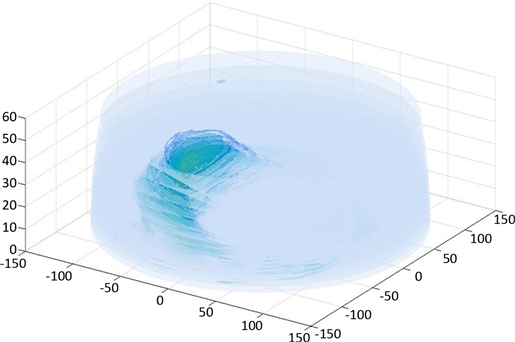Figure 3. Radar data cone model

2.2. 立方体网格插值算法

D点的回波强度来自于以该点为公共点的八个立方体内数据采样点的回波强度的加权值。实际上所有有效的数据采样点在以D点为球心、半径为R的球体内，D的回波强度值为计算该球体内所有采集点的气象回波强度值的加权平均值。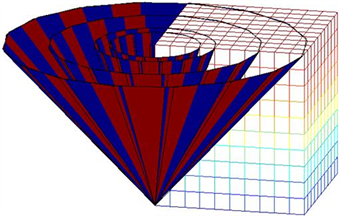Figure 4. Embedding conical data into cube mesh figureFigure 5. Interpolation point D and unit cube DiagramFigure 6. The relation between interpolation point D and sampling Points

$d\left(x,y,z\right)=\frac{\underset{i=1}{\overset{n}{\sum }}\left[q\left(i\right)×{d}_{i}\left({x}_{i},{y}_{i},{z}_{i}\right)\right]}{\underset{i=1}{\overset{n}{\sum }}q\left(i\right)}$ (1)

$q\left(i\right)=\frac{1}{\Delta {l}^{\lambda }}-1,\text{\hspace{0.17em}}\text{\hspace{0.17em}}\left(\lambda >0\right)$ (2)

λ是一个与权值分配有关的参数，Δl表示采样点与标准立方体顶点D之间的距离。λ决定了权值分配与Δl之间的联系。本文中取λ = 1，当λ = 1时，权值大小与Δl之间存在反比关系。

$\Delta l=\sqrt{\Delta {x}^{2}+\Delta {y}^{2}+\Delta {z}^{2}}$ (3)

2.3. 结合MC算法实现三维重建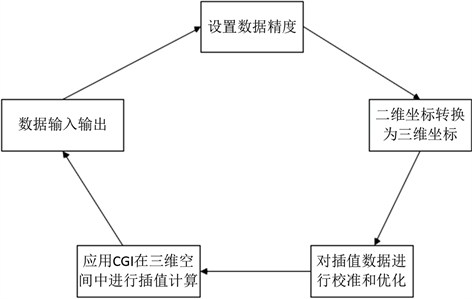Figure 7. CGI algorithm flow chartFigure 8. Three-dimensional radar data field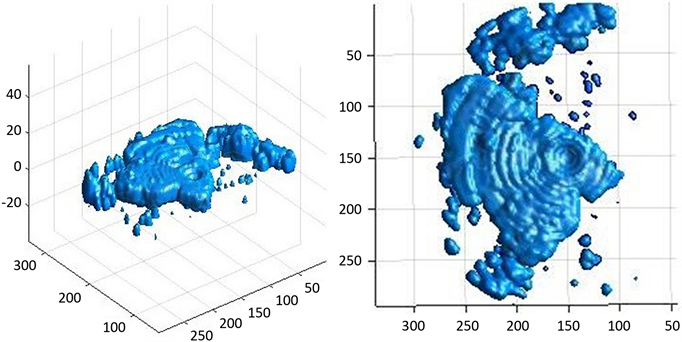Figure 9. 3-D reconstructed cloud results

3. 实验结果分析

① 不同回波强度值的重建结果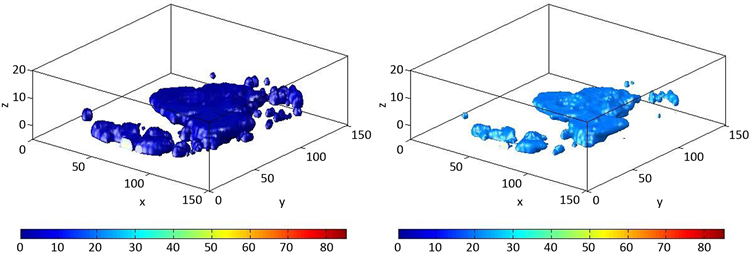(a) Echo intensity value ≥ 0 dBZ(b) Echo intensity value ≥ 20 dBZ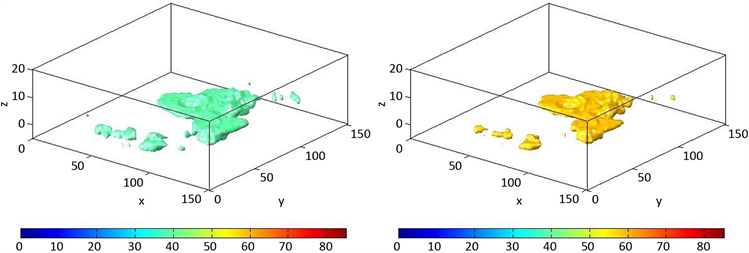(c) Echo intensity value ≥ 40 dB Z(d) Echo intensity value ≥ 60 dBZ

Figure 10. Reconstruction results of different echo intensity values

② 不同剖面的重建结果

③ 不同时刻的重建结果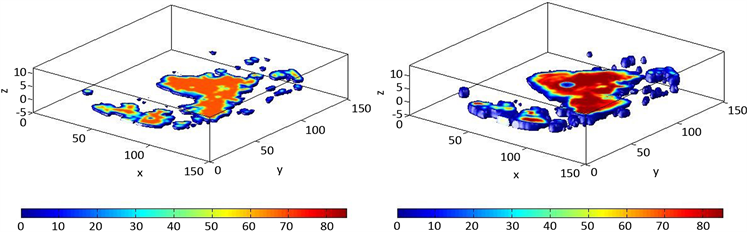(a) Height =2 km (b) Height = 4 km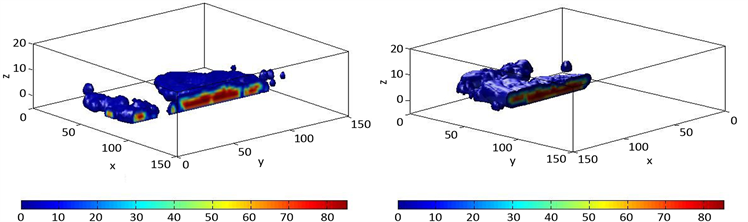(c) YOZ平面垂直剖分 (d) XOZ平面垂直剖分

Figure 11. Reconstruction results of different sections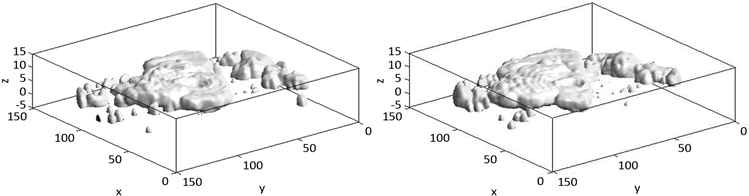(a) 00:00时刻 (b) 00:59时刻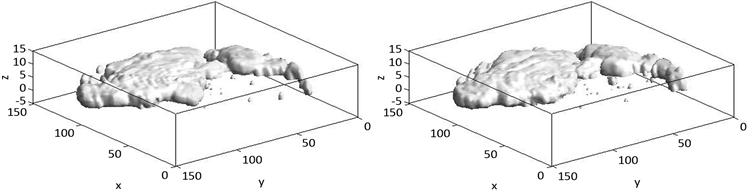(c) 01:59时刻 (d) 02:58时刻

Figure 12. Reconstruction and rendering results at different times

4. 总结

  Ernvik, A. (2002) 3D Visualization of Weather Radar Data. Department of Electrical Engineering, Linkoping.  Ru, Y. (2007) Volumetric Visualization of NEXRAD Level II Doppler Weather Data from Multiple Sites.  Denham, M., Lamperti, E. and Areta, J. (2018) Weather Radar Data Processing on Graphic Cards. Journal of Supercomputing, 74, 868-885. https://doi.org/10.1007/s11227-017-2166-8  王轩, 路明月, 郑建琴, 等. 雷达数据的多维可视化[J]. 图学学报, 2017, 38(4): 497-503.  刘岩, 刘建朝, 孙海燕. 天气雷达数据三维可视化技术及应用[J]. 气象灾害防御, 2014, 21(4): 41-43.  路明月, 毛远翔, 孟昭林, 等. 面向web三维应用的气象雷达矢量剖面生成[J]. 科学技术与工程, 2019, 19(1): 166-171.